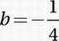# SAT Math Multiple Choice Question 705: Answer and Explanation

### Test Information

Question: 705

15. If f(x) = (x2)-2b and f(3) = 3, what is the value of b?

• A.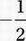• B.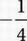• C.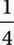• D.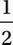Explanation:

B

f(3) = (32)-2b = 3

Exponential Law #8

(from Chapter 9, Lesson 9):

3-4b = 31

Exponential Law #10

(from Chapter 9, Lesson 9)

-4b = 1

Divide by -4: